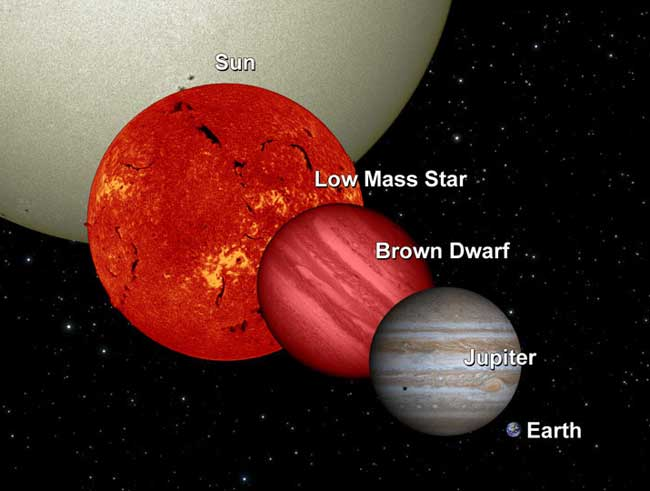# National Aeronautics and Space Administration

## How Many Stars in the Milky Way?

Recently I was asked to help someone answer the question of how many stars are in the Milky Way – that there were differing answers out there, and which was the right one?

This question turns out to have a really interesting (and possibly frustrating?) answer. And the answer is that we really don’t know. We can make estimates, but there isn’t a firm, solid, 100% answer.

Why is this? Well, first off, there isn’t a way to simply count the number of stars in the Milky Way individually – that’s where the estimates come in. To make an estimate, we have to calculate the mass of our galaxy, and then the percentage of that mass that is made up of stars.

Then we have to decide what the mass of an average star is so we can calculate the number of stars in the galaxy. This is not trivial either – you could say our Sun is an average sized star, which would give you one estimate for the number of stars in the galaxy. But our Sun may not really be typical – there are a lot of much lower-mass stars out there. Using a low-mass red dwarf as an average-mass star will give you a totally different answer for the total number of stars in our galaxy.This diagram shows a brown dwarf in relation to Earth, Jupiter, a low-mass star, and the sun. Credit: NASA

It’s kind of like if you had a 10 pound bag. You can see in the top of the bag and can tell that there are coins there, mixed in with candy, dried beans, screws, rice, and other things you can’t see, but that must be heavy enough to make the bag weigh 10 pounds. Now try to calculate how many coins are in that bag – it’s hard to do because you can only really count the coins you can see – so you have to figure out if the contents of the bag that you can see is representative of the whole of the bag. Are coins evenly distributed throughout? Let’s say you can say with relative confidence that coins make up 10% of mass of the bag, or one pound. How many coins are there total? Well, you can see pennies and quarters and nickels, and each type of coin has a different mass and size. If you picked the quarter as being the average mass of a single coin, you might get one answer for the total number of coins. But if you picked the penny, which is lighter, you’d have a higher total number of coins because there are more pennies to the pound than quarters.

There are different models for estimating the number of stars in the Milky Way and the answers they give differ depending on what is used as the average mass of a star. The most common answer seems to be that there are 100 billion stars in the Milky Way on the low-end and 400 billion on the high end. But I’ve seen even higher numbers thrown around.

For more reading on the methods scientists use to calculate things like the mass and composition of our galaxy, here are a few resources for you.

Tags: ,